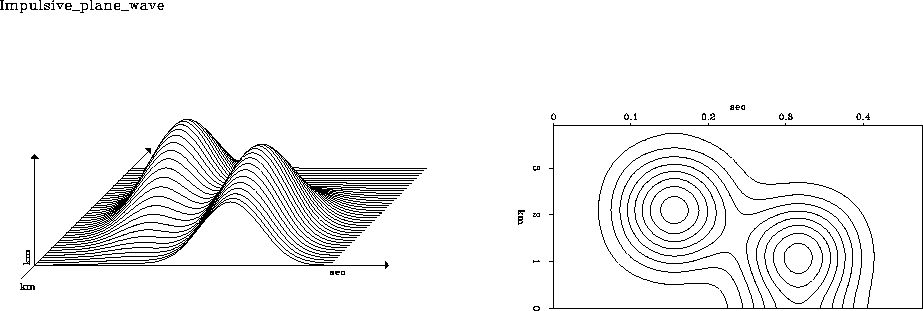Next: Speed of 2-D Gaussian Up: SMOOTHING IN TWO DIMENSIONS Previous: Tent smoothing

## Gaussian mounds

In Figure 12 we see the result of applying tent smoothing twice.mound
Figure 12
Two impulses in two dimensions filtered twice on each axis with a triangle function. Left: bird's-eye view. Right: contours of constant altitude z.

Notice that the contours, instead of being diamonds and rectangles, have become much more circular. The reason for this is briefly as follows: convolution of a rectangle with itself many times approachs the limit of a Gaussian function. (This is a well-known result called the ``central-limit theorem.'' It is explained in section.) It happens that the convolution of a triangle with itself is already a good approximation to the Gaussian function z(x)= e-x2. The convolution in y gives z(x,y)=e-x2-y2= e-r2, where r is the radius of the circle. When the triangle on the 1-axis differs in width from the triangle on the 2-axis, then the circles become ellipses.Next: Speed of 2-D Gaussian Up: SMOOTHING IN TWO DIMENSIONS Previous: Tent smoothing
Stanford Exploration Project
10/21/1998